# Generalized Linear Models

Based on the “Statistical Consulting Cheatsheet” by Prof. Kris Sankaran

Linear models provide the basis for most inference in multivariate settings. We won’t even begin to try to cover this topic comprehensively. There are entire course sequences that only cover linear models. But, we’ll try to highlight the main regression-related ideas that are useful to know during consulting.

This section is focused more on the big-picture of linear regression and when we might want to use it in consulting. We defer a discussion of inference in linear models to the next section.

There are three steps in the analysis of data using a (Generalized) Linear Model: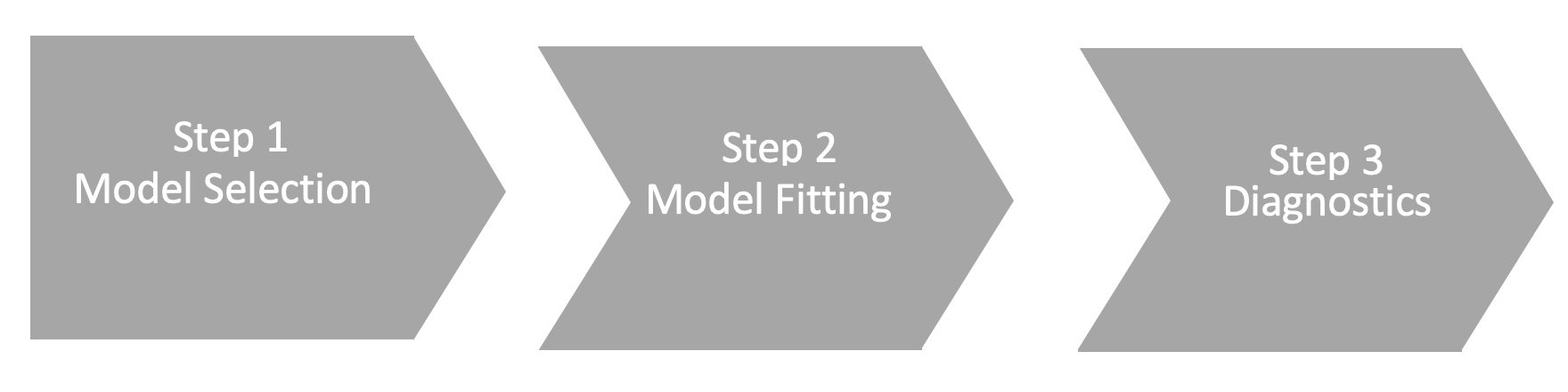Figure: the three steps in GLM analysis.

• Step 1: Model Selection, which involves determining which GLM to choose.
• Step 2: Model Fitting, which is the actual process of fitting the model to your data.
• Step 3: Diagnostics, which involves making sure that your model is a good fit and produces quality estimates

Depending on the type of data that you have at-hand, you might opt for:

• Linear Regression, if your output $$y$$ is a continuous variable.
• Logistic Regression, if your output $$y$$ is a binary variable (eg 0/1).
• Multinomial Regression, if your output $$y$$ is a categorical variable with more than 2 categories (eg 0/1/2).
• Ordinal Regression, if your output $$y$$ is a categorical variable with more than 2 categories (eg 0/1/2), and there is a natural ordering of the classes (eg 0<1<2) — this is appropriate when the output is a survey scale for instance.
• Poisson Regression, if your output $$y$$ consists of count data.

## 1. Linear regression

### 1.1. The Model

Linear regression learns a linear function between covariates and a response, and is popular because there are well-established methods for performing inference for common hypothesis.

• Generally, model-fitting procedures suppose that there is a single variable $$Y$$ of special interest. The goal of a supervised analysis is to determine the relationship between many other variables (called covariates), $$X_1, \cdots X_p$$ and $$Y$$ . Having a model $$Y = f(X_1, \cdots X_p)$$ can be useful for many reasons, especially (1) improved scientific understanding (the functional form of f is meaningful) and (2) prediction, using $$f$$ learned on one set of data to guess the value of $$Y$$ on a new collection of $$X$$’s.

•  Linear models posit that the functional form $$f$$ is linear in the $$X_1, \cdots X_p$$. This is interpreted geometrically by saying that the change in $$Y$$ that occurs when $$X_j$$ is changed by some amount (and all other covariates are held fixed) is proportional to the change in $$X_j$$ . E.g., when $$p = 1$$, this means the scatterplot of $$X_1$$ against $$Y$$ can be well-summarized by a line.  A little more formally, we suppose we have observed samples indexed by $$i$$, where $$x_i \in \mathbb{R}^p$$ collects the covariates for the $$i$$th sample, and $$y_i$$ is the associated observed response. A regression model tries to find a parameter $$\beta \in \mathbb{R}^p$$ so that

$Y_i + x_i^T \beta +\epsilon_i$

is plausible, where $$i$$ are drawn i.i.d. from $$N(0, \sigma^2)$$.  for some (usually unknown) $$\sigma^2$$. The fitted value for $$\beta$$ after running a linear regression is denoted $$\hat{\beta}$$ .

• Compared to other forms of model fitting, a major advantage of of linear models is that inference is usually relatively straightforwards { we can do tests of significance / build confidence intervals of the strength of association across different $$X$$’s as well as comparison between models with different sets of variables.  The linear assumption is not well-suited to binary, count, or categorical responses $$Y$$ , because it the model might think the response $$Y$$ belongs to some range that’s not even possible (think of extrapolating a linear in a scatterplot when the y-axis values are all between 0 and 1). In these situations, it is necessary to apply generalized linear models (GLMs) (GLMs). Fortunately, many of the ideas of linear models (methods for inference in particular) have direct analogs in the GLM setting.

### When is linear regression useful in consulting?

• In a consulting setting, regression is useful for understanding the association between two variables, controlling for many others. This is basically a rephrasing of point (2) above, but it’s the essential interpretation of linear regression coecients, and it’s this interpretation that many research studies are going after.

• Sometimes a client might originally come with a testing problem, but might want help extending it to account for additional structure or covariates. In this setting, it can often be useful to propose a linear model instead: it still allows inference, but it becomes much easier to encode more complex structure.

### What are some common regression tricks useful in consulting?

• Adding interactions: People will often ask about adding interactions in their regression, but usually from an intuition about the non-quantitative meaning of the word “interaction.” It’s important to clarify the quanti- tative meaning: Including an interaction term between $$X_1$$ and $$X_2$$ in a regression of these variables onto $$Y$$ means that the slope of the relation- ship between $$X_1$$ on $$Y$$ will be different depending on the value of $$X_2$$. For example, i f$$X_2$$ can only take on two values (say, A and B), then the relationship between $$X_1$$ and $$Y$$ will be linear with slope $$\beta_{1A}$$ in the case that $$X_2$$ is A and $$\beta_{1B}$$ otherwise11. When $$X_2$$ is continuous, then there is a continuum of slopes depending on the value of $$X_2: \beta_1 + \beta_{1\times2}X_2$$. See Figure 5 for a visual interpretation of interactions. 
• Introducing basis functions: The linearity assumption is not as restrictive as it seems, if you can cleverly apply basis functions. Basis functions are functions like polynomials (or splines, or wavelets, or trees…) which you can mix together to approximate more complicated functions, see the figure.

Linear mixing can be done with linear models. To see why this is potentially useful, suppose we want to use time as a predictor in a model (e.g., where $$Y$$ is number of species j present in the sample), but that species population doesn’t just increase or decrease lin- early over time (instead, it’s some smooth curve). Here, you can introduce a spline basis associated with time and then use a linear regression of the response onto these basis functions. The fitted coecients will define a mean function relating time and the response. 

• Derived features: Related to the construction of basis functions, it’s often possible to enrich a linear model by deriving new features that you imagine might be related to $$Y$$ . The fact that you can do regression onto variables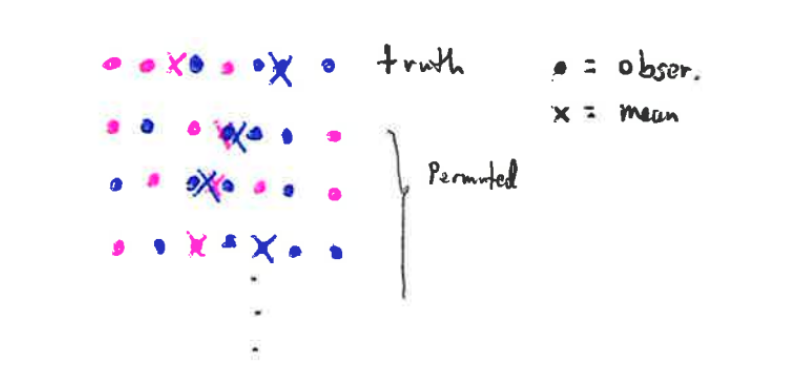Figure 5: In the simplest setting, an interaction between a continuous and binary variable leads to two different slopes for the continuous variable. Here, we are showing the scatterplot ( x_{i1}; y_i ) pairs observed in the data. We suppose there is a binary variable that has also been measured, denoted x_{i2}\$, and we shade in each point according to its value for this binary variable. Apparently, the relationship between x_1 and y depends on the value of y (when in the pink group, the slope is less. This can exactly be captured by introducing an interaction term between x_1 and x_2. In cases where x_2 is not binary, we would have a continuous of slopes between x_1 and y – one for each value of x_2. that aren't just the ones that were collected originally might not be obvious to your client. For example, if you were trying to predict whether someone will have a disease based on time series of some lab tests, you can construct new variables corresponding to the \slope at the beginning," or \slope at the end" or max, or min, ... across the time series. Of course, deciding which variables might actually be relevant for the regression will depend on domain knowledge.  One trick --- introducing random effects --- is so common that it gets it's own section. Basically, it's useful whenever you have a lot of levels for a particular categorical vector..

### 1.2. Diagnostics

How can you assess whether a linear regression model is appropriate? Many types of diagnostics have been proposed, but a few of the most common are:

• Look for structure in residuals: According to equation 1, the amount that the predictions $$\hat{y}_i = x_i^T\beta$$ is off from $$y_i$$ (this difference is called the residual $$r_i = \hat{y}_i - y_i$$) should be about i.i.d. $$N(0, \sigma^2)$$. Whenever there is a systematic pattern in these residuals, the model is misspecifed in some way. For example, if you plot the residuals over time and you find clumps that are all positive or negative, it means there is some unmeasured phenomena associated with these time intervals that influences the average value of the responses. In this case, you would define new variables for whether you are in one of these intervals, but the solution differs on a case- by-case basis. Other types of patterns to keep an eye out for: nonconstant spread (heteroskesdasticity), large outliers, any kind of discreteness (see Figure 7).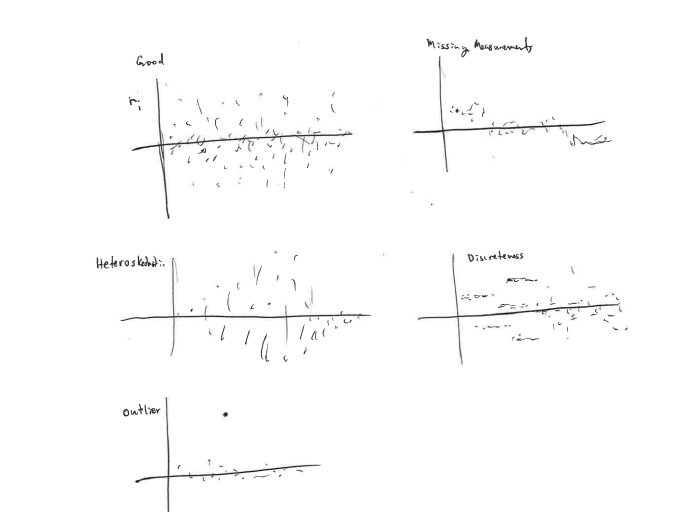Figure 7: Some of the most common types of \structure" to watch out for in residual plots are displayed here. The top left shows how residuals should appear { they look essentially i.i.d. N (0; 1). In the panel below, there is nonconstant variance in the residuals, the one on the bottom has an extreme outlier. The panel on the top-right seems to have means that are far from zero in a structured way, while the one below has some anomalous discreteness. leverage, you might consider throwing them out. Alternatively, you could consider a robust regression method.

• Make a qq-plot of residuals: More generally than simply finding large outliers in the residuals, we might ask whether the residuals are plausibly drawn from a normal distribution. qq-plots give one way of doing this – more often than not the tails are heavier than normal. Most people ignore this, but it can be beneficial to consider e.g. robust regression or considering logistically (instead of normally) distributed errors.

• Calculate the leverage of different points: The leverage of a sample is a measure of how much the overall fit would change if you took that point out. Points with very high leverage can be cause for concern { it’s bad if your fit completely depended on one or two observations only { and these high leverage points often turn out to be outliers. See Figure 8 for an example of this phenomenon. If you find a few points have very high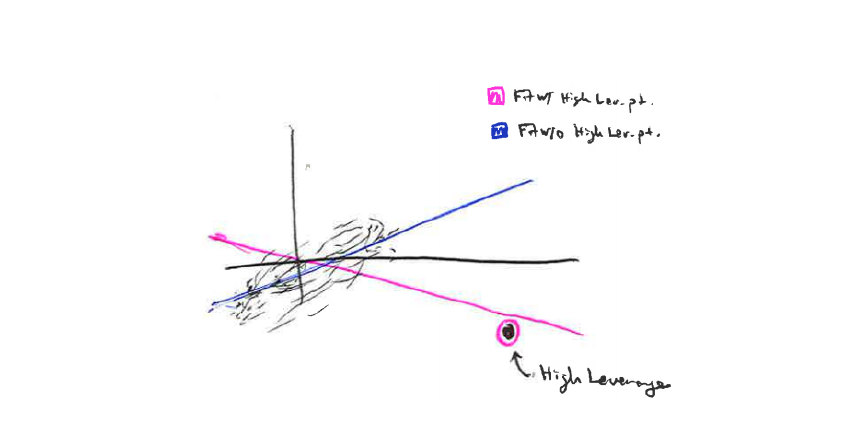Figure 8: The leverage of a sample can be thought of as the amount of influence it has in a fpt. Here, we show a scatterplot onto which we fit a regression line. The cloud near the origin and the one point in the bottom right represent the observed samples. The blue line is the regression fit when ignoring the point on the bottom right, while the pink line is the regression including that point. Evidently, this point in the bottom right has very high leverage – in fact, it reverses the sign of the association between X and Y . This is also an example of how outliers (especially outliers in the X direction) can have very high leverage.

•  Simulate data from the model: This is a common practice in the Bayesian community (‘‘posterior predictive checks”), though I’m not aware if people do this much for ordinary regression models. The idea is simple though: draw samples from $$N(x_i^T \hat{\beta}, \hat{\sigma}^2)$$ and see whether the new $$y_i$$’s look comparable to the original $$y_i$$’s. Characterizing the ways they don’t match can be useful for modifying the model to better fit the data.

## 2 - Logistic regression

### 2.1. The Model

Logistic regression is the analog of linear regression that can be used whenever the response $$Y$$ is binary (e.g., patient got better, respondent answered “yes,” email was spam).

In linear regression, the response $$y$$ are directly used in a model of the form $$y_i = x^T_i\beta+ \epsilon$$.

In logistic regression, we now want a model between the $$x_i$$ and the unknown probabilities of the two classes when we’re at $$x_i$$: $$p(x_i)$$ and $$1 - p (x_i$$).  The observed value of $$y_i$$ corresponding to $$x_i$$ is modeled as being drawn from a coin flip with probability $$p (x_i)$$.  If we had fit an ordinary linear regression model to the $$y_i$$, we might get fitted responses $$\hat{y}_i$$ outside of the valid range [0; 1], which in addition to being confusing is bad modeling.

The goal Logistic regression instead models the log-odds transformation to the $$p (x_i)$$. Concretely, it assumes the model:

$$p (y_1, y_2, \cdots, y_n) = \prod_{i=1}^n p_\beta(x_i)^{1_{y_i=1}} (1- p_\beta(x_i))^{1_{y_i=0}}$$ where we are approximating $$p(x_i) \approx p_\beta(x_i) = \frac{1}{1+ e^{-x_i^T\beta}}$$.

• This is equivalent to saying that each observation $$y_i$$ is sampled from a Bernouilli whose probability is a function of $$x_i$$: $$y_i \sim \text{Bernouilli}( p_\beta(x_i) )$$. ogistic regression fits the parameter $$\beta$$ to maximize the likelihood defined in the model above .
• An equivalent reformulation of the assumption is that $$\log(\frac{ p_\beta(x_i) }{1- p_\beta(x_i) }) = x_i^T \beta$$ i.e. the log-odds are approximately linear.
• Out of the box, the coecients fitted by logistic regression can be difficult to interpret. Perhaps the easiest way to interpret them is in terms of the relative risk, which gives an analog to the usual linear regression interpret “when the jth feature goes up by one unit, the expected response goes up by $$\beta_j$$.” First, recall that the relative risk is defined as $$r = \frac{\mathbb{P}[Y=1|X] }{\mathbb{P}[Y=0|X]}$$ which in logistic regression is approximated by $$e^{x_i^T\beta}$$. If we increase the jth coordinate of $$x_i$$ (i.e., we replace $$x_i \leftarrow x_i +\delta$$ ), then this relative risk becomes $$r = e^{x_i^T\beta} e^{\delta^T\beta}$$ The interpretation is that the relative risk got multiplied by $$e^{\beta_j}$$ we increased the jth covariate by one unit.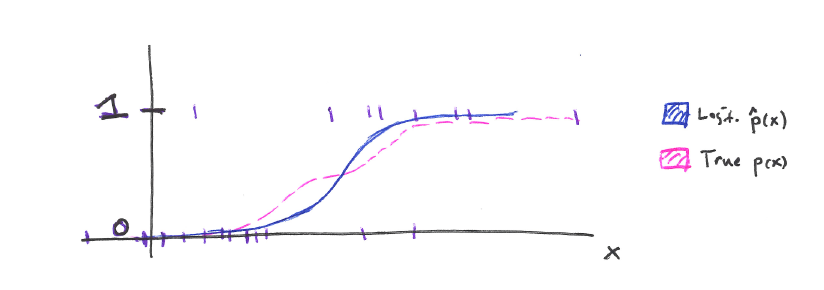Figure 9: An example of the type of approximation that logistic regression makes. The x-axis represent the value of the feature, and the y-axis encodes the binary 0 / 1 response. The purple marks are observed (xi; yi) pairs. Note that class 1 becomes more common when x is large. The pink line represents the "true" underlying class probabilities as a function of x, which we denote as p (x). This doesn't lie in the logistic family 1/(1+exp(-xB) , but it can be approximated by a member of that family, which is drawn in blue (this is the logistic regression fit).

### 2.2 Diagnostics

While you could study the differences $$y_i - \hat{p}(x_i)$$, which would be analogous to linear regression residuals, it is usually more informative to study the Pearson or deviance residuals, which upweight small differences near the boundaries 0 and 1. These types of residuals take into account structure in the assumed bernoulli (coin-flipping) sampling model.  For ways to evaluate the classification accuracy of logistic regression models, see the section on Prediction Evaluation and for an overview of formal inference, see the [section Inference in GLM].

## 3 - Multinomial regression

Multinomial regression is a generalization of logistic regression for when the response can take on one of $$K$$ categories (not just $$K = 2$$).  Here, we want to study the way the probabilities for each of the $$K$$ classes varies as x varies: $$p (y_i = j|x_i)$$ for each $$k = 1 \cdots K$$ Think of the responses $$y_i$$ as observations from a K-sided dice, and that different faces are more probable depending on the associated features xi.  The approximation of logisitc regression is replaced with

$p(y_i = k |c_i) \approx p_W( y_i=k|x_i )= \frac{\exp(w_k^Tx_i)}{\sum_k \exp(w_k^Tx_i)}$

where the parameters $$w_1\cdots;w_K$$ govern the relationship between xi and the probabilities for different classes.  As is, this model is not identifiable (you can increase one of the wks and de- crease another, and end up with the exact same probabilities $$p _W(y_i = j|x_i)$$.

To resolve this, one of the classes (say the Kth, this is usually chosen to be the most common class) is chosen as a baseline, and we set $$w_K = 0$$.  Then the $$w_k$$s can be interpreted in terms of how a change in $$x_i$$ changes the probability of observing $$k$$ relative to the baseline $$K$$. That is, suppose we increase the $$j$$th variable by one unit, so that $$x_i \leftarrow x_i + \delta_j$$ . Then, the relative probability against class K changes according to $$p(y_i = k |c_i) \approx p_W(y_i=k|x_i )= \frac{\exp(w_k^Tx_i)}{\sum_k \exp(w_k^Tx_i).}$$

## 3 - Ordinal regression

Sometimes we have $$K$$ classes for the responses, but there is a natural ordering between them. For example, survey respondents might have chosen one of 6 values along a likert scale. Multinomial regression is unaware of this additional ordering structure – a reasonable alternative in this setting is ordinal regression.

• The basic idea for ordinal regression is to introduce a continuous latent variable $$z_i$$ along with $$K-1$$ “cutpoints” $$\gamma_1, \cdots, \gamma_{K-1}$$ which divides the real line into $$K$$ intervals. When $$z_i$$ lands in the $$k$$th of these intervals, we observe $$y_i = k$$.
• Of course, neither the $$z_i$$’s nor the cutpoints $$\gamma_k$$ are known, so they must be inferred. This can be done using the class frequencies of the observed $$y_i$$s though (many $$y_i = k$$ means the $$k$$th bin is large).
• To model the influence of covariates $$p (y_i = k|x_i)$$, we suppose that $$x_i = \beta^Tx_i +\epsilon_i$$. When $$\epsilon_i$$ is Gaussian, we recover ordinal probit regression, and when $$\epsilon_i$$ follows the logistic distribution we recover ordinal logistic regression.  An equivalent formulation of ordinal logistic regression models the cumulative logits” as linear
$\log(\frac{p(y_i \leq k) }{1 - p(y_i \leq k)} ) = \alpha_k + \beta^T x_i.$

Here, the $$\alpha_k$$’s control the overall frequencies of the $$K$$ classes, while $$\beta$$ controls the influence of covariates on the response. + Outside of the latent variable interpretation, it’s also possible to under- stand $$\beta$$ in terms of relative risks. In particular, when we increase the $$j$$th coordinate by 1 unit, $$x_i \leftarrow x_i + \delta_j$$ , the odds of class $$k$$ relative to class k-11gets multiplied by $$\exp(\beta_j)$$, for every pair of neighboring classes $$k-1$$ and $$k$$.

## 4. Poisson regression

### 4.1. The Model

Poisson regression is a type of generalized linear model that is often applied when the responses $$y_i$$ are counts (i.e., $$y_i \in \{ 0, 1, \cdots \}$$.  As in logistic regression, one motivation for using this model is that using ordinary logistic regression on these responses might yield predictions that are impossible (e.g., numbers below zero, or which are not integers).  To see where the main idea for this model comes from, recall that the Poisson distribution with rate $$\lambda$$ draws integers with probabilities:

$\mathbb{P}_{\lambda} [y=k |\lambda] = \frac{\lambda^k e^{-\lambda}}{k!}$

The idea of Poisson regression is to say that the different $$y_i$$ are drawn Poissons with rates that depend on the associated $$x_i$$.

More specifically, we assume that the data have a joint likelihood:

$p(y_1, y_2, \cdots) = \prod_{i=1}^n \frac{\lambda(x_i)^{y_i} e^{-\lambda(x_i)}}{y_i!}$

and that the log of the rates are linear in the covariates:

$\log(\lambda(x_i)) = x_i^T \beta$

(modeling the logs as linear makes sure that the actual rates are always nonnegative, which is a requirement for the Poisson distribution).

We think of different regions of the covariate space as having more or less counts on average, depending on this function  $$\lambda(x_i)$$. Moving from xi to $$x_i + \delta_j$$ multiplies the rate from  $$\lambda(x_i)$$ to $$\exp(\beta_j)  \lambda(x_i).$$

### 4-2 Diagnostics.

As in logistic regression, it makes more sense to consider the deviance residuals when performing diagnostics.

In particular, a deficiency in Poisson regression models (which often motivates clients to show up to consulting) is that real data often exhibit overdipersion with respect the assumed Poisson model. The issue is that the mean and variance of counts in a Poisson model are tied together: if you sample from then the mean and variance of the associated counts are both $$\lambda$$. In real data, the variance is often larger than the mean, so while the Poisson regression model might do a good job approximating the mean  $$\lambda(x_i)$$ at $$x_i$$, the observed variance of the $$y_i$$ near $$x_i$$ might be much larger than $$\lambda (x_i)$$. This motivates the methods in following subsection .

### 4.3- Accounting for overdispersion : Pseudo-Poisson and Negative Binomial regression

Pseudo-Poisson and negative binomial regression are two common strategies for addressing overdispersion in count data. In the pseudo-Poisson setup, a new parameter ‘ is introduced that sets the relative scale of the variance in comparison to the mean: $$\mathbb{V}\text{ar} (y) = \mathbb{E}[y]$$. This is not associated with any real probability distribution, and the associated likelihood is called a pseudolikelihood. However, ‘ can be optimized along with from the usual Poisson regression setup to provide a maximum pseudolikelihood estimate. In negative binomial regression, the Poisson likelihood is abandoned alto- gether in favor of the negative binomial likelihood. Recall that the negative bi- nomial (like the Poisson) is a distribution on nonnegative counts $$\{0, 1, 2,\cdots \}$$. It has two parameters, p and r, $$\mathbb{P}[y=k] ={ k+r-1 \choose k} p^k (1-p)^r$$ which can be interpreted as the number of heads that appeared before seeing $$r$$ tails, when flipping a coin with probability $$p$$ of heads. More important than the specific form of the distribution is the fact that it has two parameters, which allow different variances even for the same mean:

$\mathbb{E}_{p,r}[y] = \frac{pr}{1-p}$ $\mathbb{V}\text{ar}_{p,r}[y] = \frac{pr}{(1-p)^2} = \mathbb{E}_{p,r}[y] + \frac{1}{2} \mathbb{E}_{p,r}[y]^2$

In particular, for small $$r$$, the variance is much larger than the mean, while for large $$r$$, the variance is about equal to the mean (it reduces to the Poisson). For negative binomial regression, this likelihood is substituted for the Poisson when doing regression, and the mean is allowed to depend on covariates. On the other hand, while the variance is no longer fixed to the mean, it must be the same across all data points. This likelihood model is not exactly a GLM (the negative binomial is not in the exponential family), but various methods for fitting it are available.

There is a connection between the negative binomial and the Poisson that both illuminates potential sources of overdispersion and suggests new algorithms for fitting overdispersed data: the negative binomial can be interpreted as a gamma mixture of Poissons.”

More specifically, in the hierarchical model:

$y | \lambda \sim \text{Poisson}(\lambda)$ $\lambda \sim \text{Gamma}(r, \frac{p}{1-p})$

which draws a Poisson with a randomly chosen mean parameter, the marginal distribution of $$y$$ is exactly NegBin$$(r, p)$$. This suggests that one reason overdis- persed data might arise is that they are actually a mixture of true Poisson subpopulations, each with different mean parameters. This is also the starting point for Bayesian inference of overdispersed counts, which fit Poisson and Gamma distributions according to this hierarchical model.